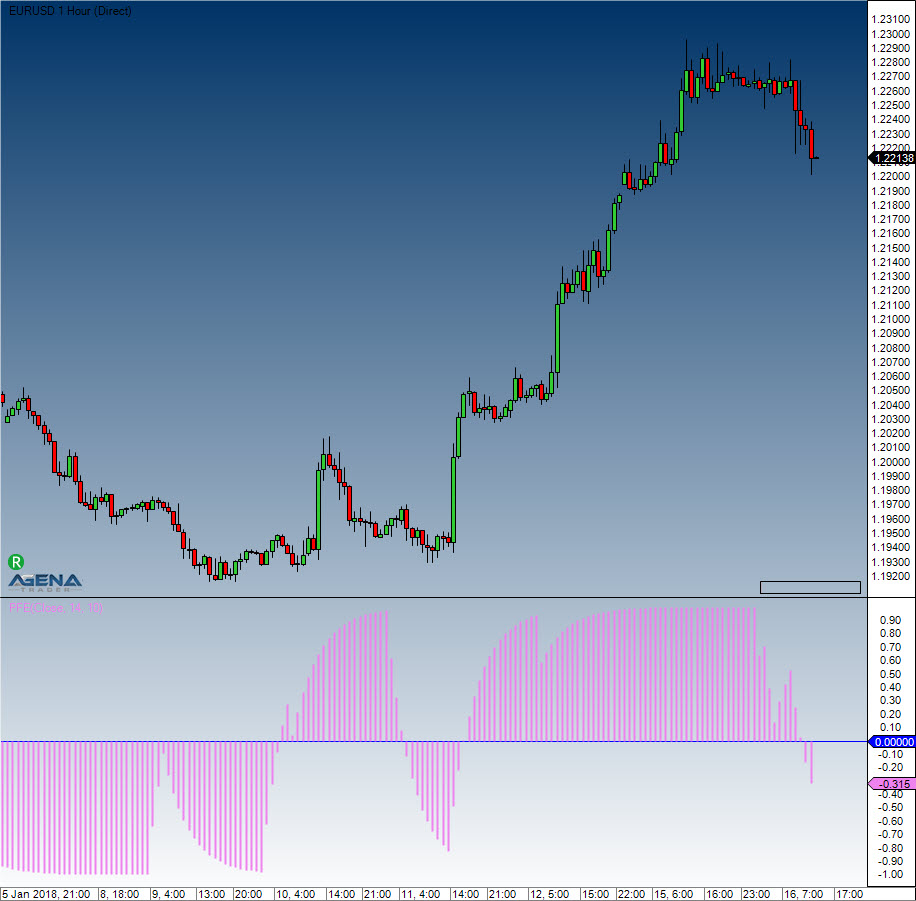Comment on page

# Polarized Fractal Efficiency (PFE)

#### Description

Hans Hannula developed the PFE, which is categorized as a momentum indicator. It uses the methods of fractal geometry and chaos theory to determine the price efficiency of the movements.

#### Interpretation

When the PFE zigzags around the zero line, no trend is present. If the PFE is equally formed and running above the zero line, the market is in an uptrend. The higher the value, the stronger the uptrend.

#### Usage

PFE(int period)
PFE(IDataSeries inSeries, int period)
PFE(int period)[int barsAgo]
PFE(IDataSeries inSeries, int period)[int barsAgo]

#### Return value

double
When using this method with an index (e.g. PFE(20)[int barsAgo] ), the value of the indicator will be issued for the referenced bar.

#### Parameters

inSeries Input data series for the indicator
period Number of bars included in the calculations

#### VisualizationPolarized Fractal Efficiency (PFE)

#### Example

//Output for the value of the Polarized Fractal Efficiency (PFE)
Print("The current value for the PFE is: " + PFE(20));